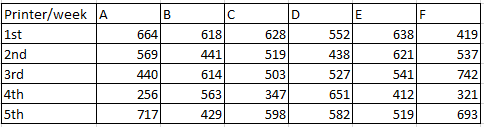### SBI PO 2017 Question 12

Instructions

Study the following table carefully and answer the questions.Question 12

# What will be the average number of pages printed by all the given printers in 4th week?

Solution

The average number of pages printed by all the given printers in 4th week will be =

$$\frac{256+563+347+651+412+321}{6} = \frac{2550}{6}$$ = $$425$$